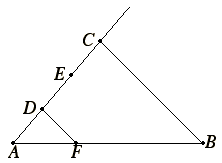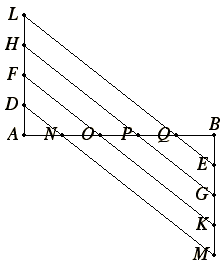# Proposition 9

To cut off a prescribed part from a given straight line.

Let AB be the given straight line.It is required to cut off from AB a prescribed part.

Let the third part be that prescribed.

I.3

Draw a straight line AC through from A containing with AB any angle. Take a point D random on AC, and make DE and EC equal to AD.

I.31

Join CB, and draw DF through D parallel to it.

VI.2

Then, since DF is parallel to a side CB of the triangle ABC, therefore, proportionally, AD is to DC as AF is to FB.

But DC is double AD, therefore FB is also double AF, therefore AB is triple of AF.

Therefore from the given straight line AB the prescribed third part AF has been cut off.

Q.E.F.

## Guide

The word “part” in this proposition means submultiple. The problem here is to divide a line AB into some given number n of equal parts, or actually, to to find just one of these parts. Euclid takes the case n = 3 in his proof.

Simson complained that proving the general case by using a specific case, the one-third part, “is not at all like Euclid’s manner.” But it is very much Euclid’s manner throughout books V and VI to prove a general numerical statement with a specific numerical value.

#### Al-Nayrizi’s constructionAbu’l-Abbas al-Fadl ibn al-Nayrizi (fl. c. 897, d. c. 922) wrote a commentary on the first ten books of the Elements. He gives another construction to divide a line AB into n equal parts. First, construct equal perpendiculars at A and B in opposite directions, mark off n – 1 equal parts on each of them, and connect the points as illustrated. The diagram shows AB divided into five equal parts. Al-Nayrizi’s construction takes considerably less work than Euclid’s. The proof that this construction is valid is about the same length as that for Euclid’s construction.

#### Use of this construction

This construction is used in a few propositions in Book XIII to find a third or a fifth of a line.